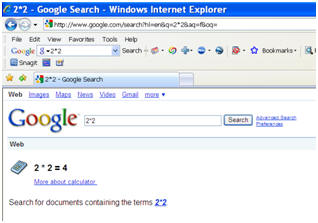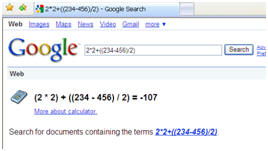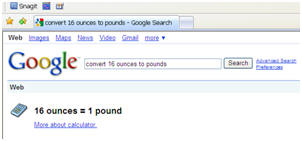1. Create a new Word document. Save it as: Last_First_calculations8B   (last two items are your grade and your teacher). Enable your drawing and pictures, then go to Tools, Options,  and add a check for paragraph marks (under View), and uncheck the drawing canvas box (under General). If you don't know how to do that, click here for help.

2. The search bar for Google will let you type in calculations. Here is an example.Here are some guidelines: Multiply is *, Divide is /, Add is +, Subtract is -   You can use parenthesis just like you would in math.
Here is an example:3. Practice a few things. You can also convert things. For instance 16 ounces to pounds

Always type the word "convert" when you do this.4. Complete the following assignments. Print the screen for each, and paste it into your Word document. Crop it similar to the examples up above.

a. Multiply 2 times 8 times 2 times 2 times 2 divided by 64.

b. Multiply 2 times 50 divided by 2 times 20

c. Convert 12 inches into centimeters

d. Convert 1000 mm into cm

e. Convert 14 pounds into grams

f. Convert 1 liter into milliliters

5. Be sure to save your document and call the teacher to grade it.